• 逻辑回归是统计学中经典的分类算法，与...1.二项逻辑回归原理及其参数的求解如下： 2.多项逻辑回归的原理 参考链接：http://www.cnblogs.com/yinheyi/p/6131262.html 参考链接：http://www.jianshu.com/
逻辑回归是统计学中经典的分类算法，与最大熵一样都是对数线性模型。两者都是以似然函数为目标函数的最优化问题。通常通过迭代算法求解，比如上篇的梯度下降法。
1.二项逻辑回归原理及其参数的求解如下：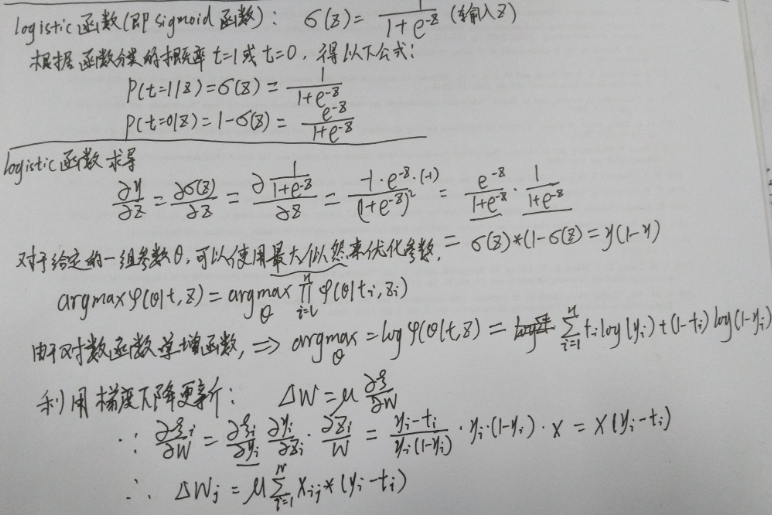2.多项逻辑回归的原理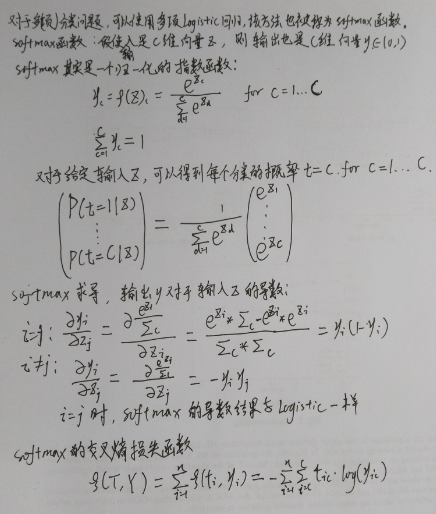参考链接：http://www.cnblogs.com/yinheyi/p/6131262.html
参考链接：http://www.jianshu.com/p/8eb17fa41164
展开全文• 本文转载多篇博客，综合了一下，组成了由基础到深入理解逻辑回归的博客。比较系统 ... 2.回归的概念：https://blog.csdn.net/alw_123/article/details/82825785 ...3.二项逻辑回归：https://blog.csdn.net...
本文转载多篇博客，综合了一下，组成了由基础到深入理解逻辑回归的博客。比较系统
1.回归的概念一：https://blog.csdn.net/alw_123/article/details/82193535
2.回归的概念二：https://blog.csdn.net/alw_123/article/details/82825785
3.二项逻辑回归：https://blog.csdn.net/pxhdky/article/details/82497304
4.多项逻辑回归：https://blog.csdn.net/huangjx36/article/details/78056375
5.模型参数估计：https://blog.csdn.net/pxhdky/article/details/83050712
展开全文算法 人工智能
• 1.逻辑回归与多项逻辑回归1.1什么是逻辑回归逻辑回归，可以说是在线性回归的基础上加上一个sigmoid函数，将线性回归产生的值归一化到[0-1]区间内。sigmoid函数如下：​ sigmoid(x)=11+e−xsigmoid(x)=\frac{1}{1+e...
1.逻辑回归与多项逻辑回归
1.1什么是逻辑回归？
逻辑回归，可以说是在线性回归的基础上加上一个sigmoid函数，将线性回归产生的值归一化到[0-1]区间内。sigmoid函数如下：
​

sigmoid(x)=11+e−x

sigmoid(x)=\frac{1}{1+e^{-x}}

[问题] ：为什么要提出逻辑回归呢？
在线性回归中，我们知道

θTX
$\theta^TX$ 就代表了分类的置信度。我们定义一个阈值

θ0
$θ_0$ , 来进行分类，但实际上，我们都知道

θTX
$θ^TX$ 的值与

θ0
$θ_0$ 的差值越大，则分类正确的概率越大，差值越小则正确概率越小。我们希望输出这样的一个概率，就需要将

θTX
$\theta^TX$ 的范围从

(−∞,+∞)
$(-\infty, +\infty)$ 限制到(0,1)中。
那么，为什么要使用sigmoid函数呢？
原因有下：
sigmoid函数输出范围为(0,1) ;sigmoid函数是一个单调递增函数，满足

θTX
$\theta^TX$越大时，输出值越大;对于二分类问题，它的概率分布满足伯努利分布，也就是 ​

{Pn=pPn=1−pn=1n=0
$\begin{cases} P_n=p& n=1 \\ P_n=1-p & n=0 \end{cases}$
这里，我们引入一个发生比

odds=p1−p
$odds=\frac{p}{1-p}$ , 做如下操作：
​ 1. 两边同时取ln,

ln(odds)=ln(p1−p)
$ln(odds)=ln(\frac{p}{1-p})$
​ 2.

logit(p)=ln(odds)=ln(p1−p)
$logit(p)=ln(odds)=ln(\frac{p}{1-p})$ 由于对于这个函数，定义域为(0,1) , 值域为(-∞，+∞)
​ 3. 取反函数，

x=ln(p1−p)e−x=1−ppp=11+e−x
$x=ln(\frac{p}{1-p})\quad e^{-x}=\frac{1-p}{p} \quad p=\frac{1}{1+e^{-x}}$ 这样，就得到了sigmoid函数。
(以上省略其他关于最大熵的原因，及相关推导)

[总结] ：
逻辑回归对于每一个样本n被分为某一类的概率，输出：

p(xn)=11+e−∑dj=1θjxnj
$p(x^n)=\frac{1}{1+e^{-\sum_{j=1}^d\theta_jx_j^n}}$
​ 用于分类时，通过采用极大似然估计进行分类，即选择类别更大的p(x)。
​
1.2什么是多项逻辑回归？
从上述可知，逻辑回归只适用于二分类问题。为了使它能扩展到多分类问题，我们将sigmoid函数，换成softmax函数。
​

softmax(x)=ei∑ck=1ek
$softmax(x)=\frac{e^i}{\sum_{k=1}^ce^k}$ （其中，ei代表第i类，c代表一共的类别数)
应用到多项逻辑回归中是：
​

p(y=i|x)=exp{ai}∑cn=1exp{an}
$p(y=i|x)=\frac{exp \{ {a_i}\}}{\sum_{n=1}^cexp\{a_n\}}$

ai=∑dj=1θijxj
$a_i=\sum_{j=1}^d \theta_{ij}x_j$
(其中，θj代表第j类的参数值，p(y=j|x)代表对于样本X，将其分为j类的概率)
[证明] ：推导出softmax函数
每一类的概率

pn
$p_n$ 取值范围在(0,1) , 而

θTX
$\theta^TX$ 的取值范围在

(−∞,+∞)
$(-\infty,+\infty)$ ，我们需要选择一个递增函数，将

θTX
$\theta^TX$ 映射到

pn
$p_n$ 。
首先，不考虑pn的取值范围为(0,1),
假设

ln(pn)=θTXpn=exp{θTX}
$ln(p_n)=\theta^TX\qquad p_n=exp\{\theta^TX\}$
再对pn进行归一化处理就可以了。

[总结] ：
多项逻辑回归，每一个样本对应分到每一类的概率：
​

p(y=i|x)=exp{ai}∑cn=1exp{an}
$p(y=i|x)=\frac{exp \{ {a_i}\}}{\sum_{n=1}^cexp\{a_n\}}$

ai=∑dj=1θijxj
$a_i=\sum_{j=1}^d \theta_{ij}x_j$
1.3如何求多项逻辑回归的模型？
1.3.1点估计
求多项逻辑回归的模型，等价于估计模型参数。
参数的估计，在统计学中，分为两类，一类是点估计；一类是区间估计。
点估计：用样本统计量来估计总体参数，因为样本统计量为数轴上某一点值，估计的结果也以一个点的数值表示，所以称为点估计。
区间估计：通过从总体中抽取的样本，根据一定的正确度与精确度的要求，构造出适当的区间，以作为总体的分布参数(或参数的函数)的真值所在范围的估计。
点估计常用方法：
矩估计法 以样本矩的某一函数，代替总体的同一函数，来构造估计量的方法。 比如，先构建一个总体的期望与参数之间的关系，然后可以用样本的均值代替总体的期望，从而求出总体的参数。最大似然估计法 当从模型总体随机抽取n组样本观测值后，最合理的参数估计量应该使得从模型中抽取该n组样本观测值的概率最大，而不是像最小二乘估计法旨在得到使得模型能最好地拟合样本数据的参数估计量。

θ=argmax(p(D|θ))=argmax(∏nm=1p(xm|θ))
$\theta =argmax(p(D|\theta))= argmax(\prod^n_{m=1} p(x_m|\theta))$最小二乘法 最小二乘法（又称最小平方法）是一种数学优化技术。它通过最小化误差的平方和寻找数据的最佳函数匹配。利用最小二乘法可以简便地求得未知的数据，并使得这些求得的数据与实际数据之间误差的平方和为最小。 ​贝叶斯估计法 贝叶斯估计，是在给定训练数据D时，确定假设空间H中的最佳假设。 最佳假设：一种方法是把它定义为在给定数据D以及H中不同假设的先验概率的有关知识下的最可能假设。

θ=argmax(p(θ|D))=argmax(p(D|θ)p(θ)p(D))
$\theta=argmax(p(\theta|D))=argmax(\frac{p(D|\theta)p(\theta)}{p(D)})$  ​ 对于逻辑回归，我们可以采用极大似然估计，也可以采用贝叶斯估计法。只不过，如果采用贝叶斯估计法，需要对θ给一个先验分布。除此之外，我们也可以采用最小二乘法。 如果我们采用极大似然估计或者贝叶斯估计法，都是需要通过梯度下降或者牛顿法等优化算法进行寻优的。而如果我们采用最小二乘法，不可以用普通最小二乘法(OLS)，而应该采用迭代加权最小二乘法(IRLS)。 ​
[问题]： 为什么不能用普通最小二乘法？
广义的最小二乘法有两种，一种是线性最小二乘法，另一种是非线性最小二乘法。
我们常说的普通最小二乘法(OLS)，就是使计算残差的误差函数最小，但是计算过程不是通过迭代，而是通过直接计算。
证明：
定义损失函数为：

L(θ)=∑mn=1(y−h(θ))2
$L(\theta)=\sum_{n=1}^m(y-h(\theta))^2$
使L(θ)最小，等同于让其偏导数为0。故有：
对于线性回归而言

∇θiL(θ)=∑mn=12(y−θ)h′(θ)
$\nabla_{\theta_{i}} L(\theta)=\sum_{n=1}^m2(y-\theta)h'(\theta)$
可以很方便的求出它的解。但是对于多项逻辑回归而言，这个偏导数的解很难求。所以不能用最小二乘法。
一个比较通俗的解释就是，本身OLS的提出，就是在高斯-马尔可夫定理的基础下，残差最小即全局最小。但是对于逻辑回归，我们的损失函数不能为平方损失函数，故而不适用OLS。
1.3.2 costfunction函数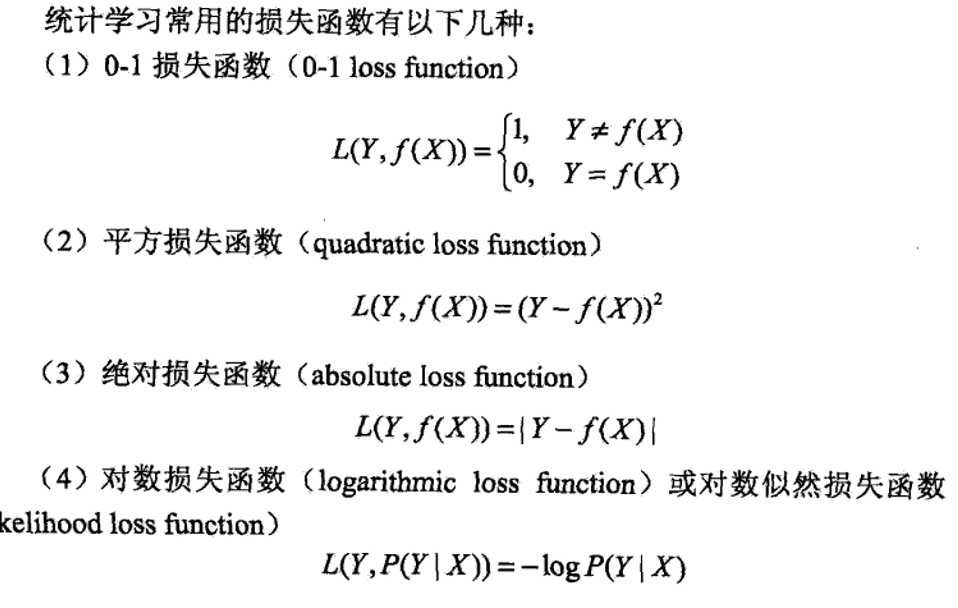(截自李航《统计学习方法》)
这里，我们的损失函数选择对数损失函数，原因有下：
逻辑回归引入softmax函数，使输出值与模型参数之间的关系不再是线性关系。此时如果选择与线性回归一样的平方损失函数，就会导致costfunction可能是非凸函数。对于非凸函数进行梯度下降法，会导致陷入局部最优情况。选择对数损失函数，会使这个函数变成凸函数。逻辑回归中概率P(Y|X)使用了指数函数。损失函数最小化通常需要对其求偏导数，直接对指数函数求导会较复杂，使用对数后，求导会简单些。逻辑回归中，损失函数若不采用对数形式，对于样本集合则有： ​

L=∏mn=1(∑cj=11{yn=j}P(yn|xn))
$L=\prod_{n=1}^m(\sum_{j=1}^{c}1\{y_n=j\}P(y_n|x_n))$  如果加上对数，则变成了

L=∏mn=1(∑cj=11{yn=j}ln(P(yn|xn)))=∑mn=1∑cj=11{yn=j}ln(P(yn|xn))
$L=\prod_{n=1}^{m}(\sum_{j=1}^{c}1\{y_n=j\}ln(P(y_n|x_n))) = \sum_{n=1}^{m}\sum_{j=1}^{c}1\{y_n=j\}ln(P(y_n|x_n))$ 累加比累乘要简单。对数函数也是单调递增函数，P(Y|X)越大，log(P(Y|X))就越大。逻辑回归模型学习的目标，就是需要使每一个样本X正确分类到类别Y的概率最大。(取负log是为了符合最小化损失函数这一理念)
（实际上，对于分类问题，输出值为概率时，基本全采用对数损失函数。对于对数损失函数的选择，还有一些观点在此省略）
由上述可得，我们的学习目标就是：

θ=argmax(∑mn=1∑cj=11{yn=j}ln(P(y=j|xn)))
$\theta = argmax(\sum_{n=1}^{m}\sum_{j=1}^{c}1\{y_n=j\}ln(P(y=j|x_n)))$
为了符合最小化损失函数这一理念：

θ=argmin(−∑mn=1∑cj=11{yn=j}ln(P(y=j|xn)))
$\theta = argmin(-\sum_{n=1}^{m}\sum_{j=1}^{c}1\{y_n=j\}ln(P(y=j|x_n)))$
(其中1{yn=j}代表示性函数，当第n个样本的分类为j时，则返回1，否则为0)
展开全文• 一、逻辑斯蒂分布/回归模型 模型描述的是一种什么样的事件或现象： 设X是连续随机变量，X服从逻辑斯蒂回归分布是指X具有下列分布函数和密度函数： 附上逻辑斯蒂分布的密度函数与分布函数，如下： 物理...
一、逻辑斯蒂分布/回归模型
模型描述的是一种什么样的事件或现象：
设X是连续随机变量，X服从逻辑斯蒂回归分布是指X具有下列分布函数和密度函数：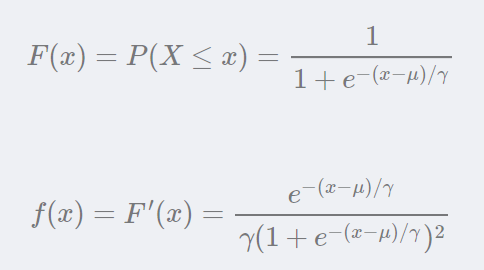附上逻辑斯蒂分布的密度函数与分布函数，如下：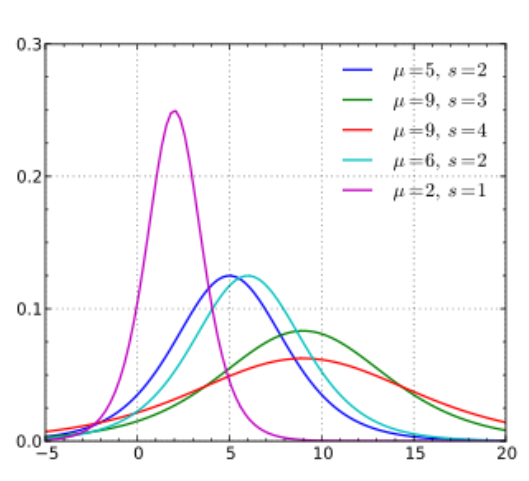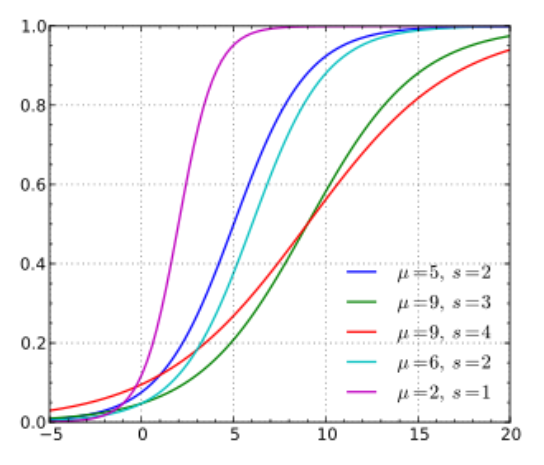物理含义，一张密度函数，一张分布函数，他们有什么物理内涵呢？为什么有些分类方法可以用这种逻辑斯蒂回归模型去做分类？而不是其它函数？下面先介绍二项逻辑斯蒂回归模型。
二、二项逻辑斯蒂回归模型
模型描述的是一种什么样的事件或现象？
二项逻辑斯蒂回归模型是一种分类模型。该模型最终形式表现为一种概率模型，这是和几何分类模型最大的区别，如SVM,KNN等。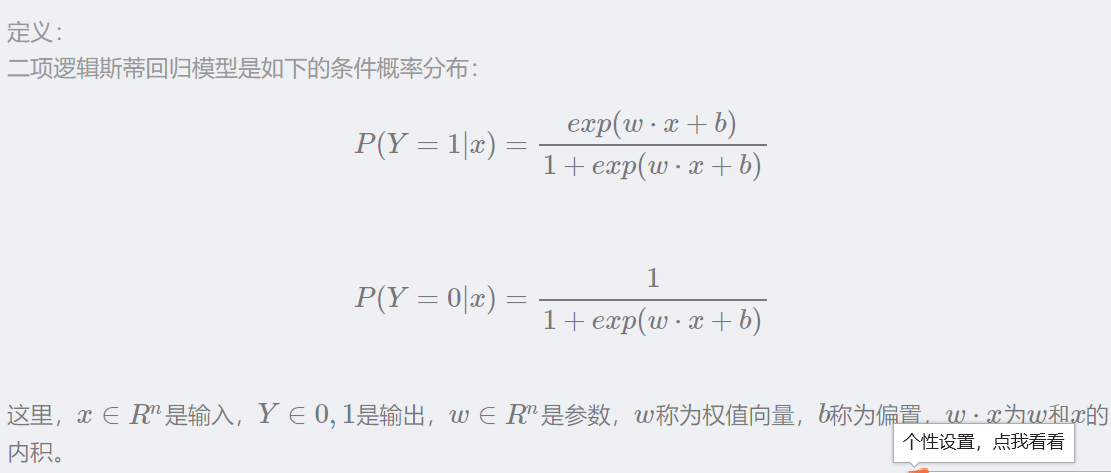三、多项逻辑斯蒂回归模型
模型描述的是一种什么样的事件或现象？
展开全文• 15_岭回归-Ridge、岭回归API、线性回归和岭回归的对别；逻辑回归、sigmoid函数、逻辑回归公式、损失函数、逻辑回归API、逻辑回归案例、逻辑回归的优缺点、逻辑回归 VS 线性回归等.pdf
• ## 逻辑回归(logistics regression)

万次阅读 多人点赞 2018-11-10 22:39:17
逻辑回归(logistics regression)  前几章分别讲了多元线性回归的推理思路和求解过程(解析解求解和梯度下降求解),文章并不以代码和公式推导过程为重点,目的是跟大家一起理解算法.前两章的内容是学习算法的基础,所以...交叉熵损失函数
• 码农 转 机器学习，logistic regression 推导过程，有启发，0基础可看懂，用颜色标注的很清楚 （修正前一个pdf问题）
• 本文介绍回归模型的原理知识，包括线性回归、多项式回归和逻辑回归，并详细介绍Python Sklearn机器学习库的LinearRegression和LogisticRegression算法及回归分析实例。进入基础文章，希望对您有所帮助。机器学习 数据分析
• 逻辑回归一般指logistic回归。logistic回归又称logistic回归分析，是一种广义的线性回归分析模型，常用于数据挖掘，疾病自动诊断，经济预测等领域。
• ## 逻辑回归

千次阅读 2019-09-20 09:38:27
然而逻辑回归，是一种名为“回归”的线性分类器器，其本质是由线性回归变化而来的，一种广泛使用于分类问题中的广义回归算法。要理理解逻辑回归从何而来，得要先从线性回归开始。线性回归是机器器学习中最简单的的...
• 线性逻辑回归 本文用代码实现怎么利用sklearn来进行线性逻辑回归的计算，下面先来看看用到的数据。 这是有两行特征的数据，然后第三行是数据的标签。 python代码 首先导入包和载入数据 写一个画图的函数，把...
• # 通过调用scipy.optimize.optimize求解逻辑斯蒂回归模型中的theta参数： import scipy.optimize as opt res = opt.minimize(fun=cost, x0=theta, args=(X, y), method='Newton-CG', jac=gradient) # theta参数存在...Python 决策边界
• 关于二项逻辑斯谛回归和多项逻辑斯谛回归的探讨，本文主要参考 MLaPP 第9章1. 指数家族在介绍逻辑斯谛回归模型之前，我们要先介绍一下指数家族。为什么要介绍指数家族呢？ 在 MLaPP 9.2.6 中证明了，指数家族是最少...
• 2逻辑回归处理的是分类，源自于y的分布：二项分布。 接着，逻辑回归的几个问题： 1、逻辑回归，为什么叫回归，却处理分类问题。 2逻辑回归怎么就是广义线性模型了？广义线性体现在哪里？ 3、逻辑回归的...广义线性模型
• ## 逻辑回归详解

千次阅读 2018-08-15 22:08:19
什么是逻辑回归逻辑回归的损失函数、梯度下降算法推导与逻辑回归的 Python 实现机器学习 Python
• 逻辑回归模型训练分类器并可视化（每一行表示一个样本，每一行有三个数值，其中前两个值代表输入属性x，最后一个值代表标签y）
•python 深度学习 机器学习 逻辑推理
• sigmoid function: ...二项逻辑回归 （binomial logistic regression model）: 多元逻辑回归（multi-nomial logistic regression model）：多分类，Y的取之集合为{1,2,...,K} softmax : ...
• 为什么叫逻辑回归？ 我们先欣赏一条S型曲线： 再来欣赏一条长得比较像、但是比较直的“曲线”： 图一叫做 logistic function，又叫做 sigmod function。图叫做单位阶跃函数。逻辑函数取值范围是(0, 1)，单位阶跃...机器学习 人工智能
• ## 二值逻辑回归

千次阅读 2018-08-07 15:26:20
逻辑回归 　在许多社会经济问题中，所研究的因变量往往只有两个可能的结果，这样的结果可以用虚拟变量来表示，虚拟变量的取值可取0和1。 　针对０－１型的因变量，我们对回归模型应该做下面的改进。 　首先...机器学习
• ## 逻辑回归详谈

千次阅读 多人点赞 2013-09-01 22:03:56
本文从数学上对逻辑回归做了一个详尽的分析，其中包括回归分析、最小二乘法、sigmoid 函数以及梯度下降等知识点的介绍。回归分析 梯度下降法 最小二乘法
• 1. 多项逻辑斯蒂回归模型 多项逻辑斯蒂回归模型（multi-...前面在逻辑斯蒂回归原理推导与求解中讲到的逻辑斯蒂回归模型是二项分类模型，用于分类。将其推广为多分类，假设离散型随机变量的取值集合是，那么多...softmax回归 机器学习
• 项逻辑回归（MNL）是一种对车辆碰撞严重性进行建模的有吸引力的统计方法，因为与其他方法（例如需要满足这些假设的判别分析）相比，该方法不需要假设正态性，线性或均方差。 此外，当将因变量转换为连续变量时，它...
• 什么是逻辑回归？ Logistic回归与多重线性回归实际上有很多相同之处，最大的区别就在于它们的因变量不同，其他的基本都差不多。正是因为如此，这两种回归可以归于同一个家族，即广义线性模型（generalizedlinear ......